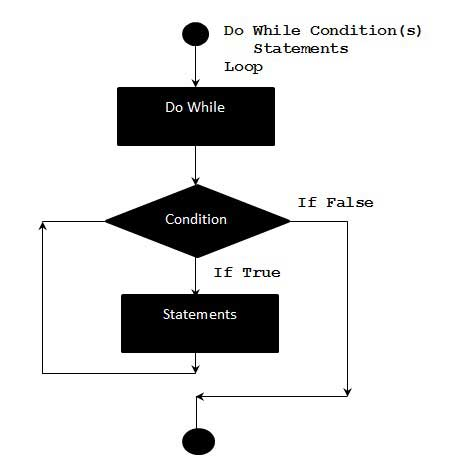# VBA - Do-While Loops

A Do…While loop is used when we want to repeat a set of statements as long as the condition is true. The condition may be checked at the beginning of the loop or at the end of the loop.

## Syntax

Following is the syntax of a Do…While loop in VBA.

```Do While condition
[statement 1]
[statement 2]
...
[statement n]
[Exit Do]
[statement 1]
[statement 2]
...
[statement n]
Loop
```

## Flow Diagram### Example

The following example uses Do…while loop to check the condition at the beginning of the loop. The statements inside the loop are executed, only if the condition becomes True.

```Private Sub Constant_demo_Click()
Do While i < 5
i = i + 1
msgbox "The value of i is : " & i
Loop
End Sub
```

When the above code is executed, it prints the following output in a message box.

```The value of i is : 1

The value of i is : 2

The value of i is : 3

The value of i is : 4

The value of i is : 5
```

## Alternate Syntax

There is also an alternate Syntax for Do…while loop which checks the condition at the end of the loop. The major difference between these two syntax is explained in the following example.

```Do
[statement 1]
[statement 2]
...
[statement n]
[Exit Do]
[statement 1]
[statement 2]
...
[statement n]
Loop While condition
```

### Example

The following example uses Do…while loop to check the condition at the end of the loop. The Statements inside the loop are executed at least once, even if the condition is False.

```Private Sub Constant_demo_Click()
i = 10
Do
i = i + 1
MsgBox "The value of i is : " & i
Loop While i < 3 'Condition is false.Hence loop is executed once.
End Sub
```

When the above code is executed, it prints the following output in a message box.

```The value of i is : 11
```
vba_loops.htm